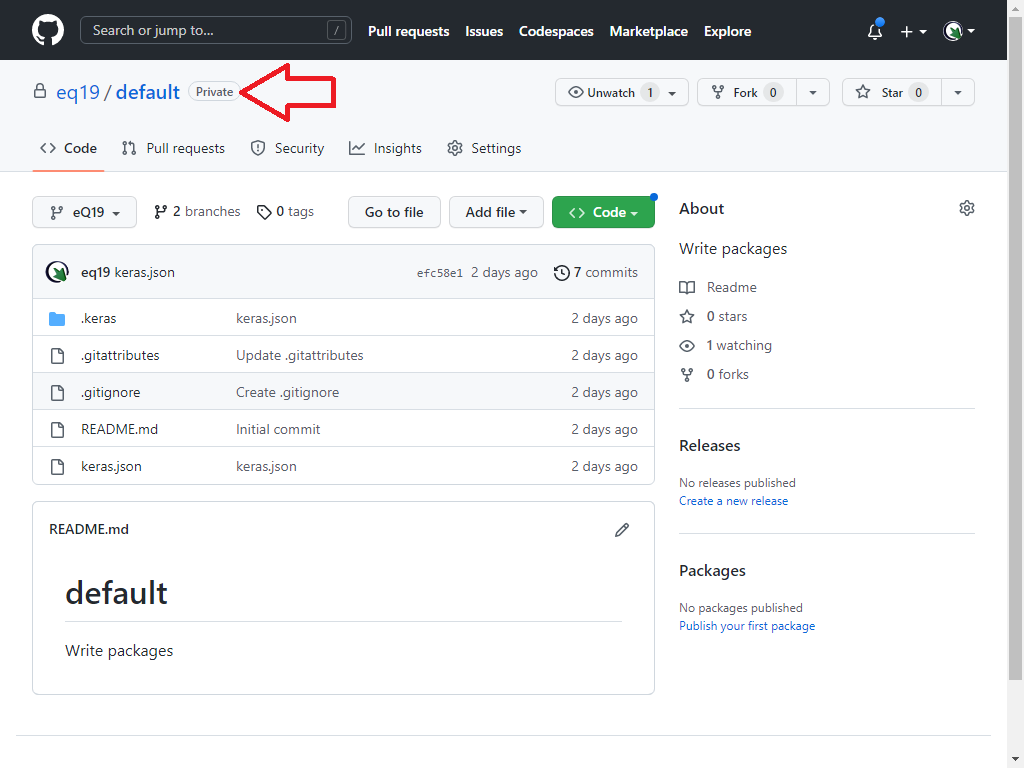# Feeding (spin 3)

As shown in The Primes Platform this cycle goes to the prime 13 then reinjected through index 13:9 . So it forms The Scheme-139 through the infinitely primes cycling. This scheme will be implemented by taking the following as the syntax algorithm: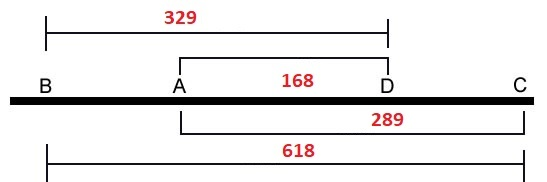The Prime Spiral Sieve possesses remarkable structural and numeric symmetries. For starters, the intervals between the prime roots (and every subsequent row or rotation of the sieve) are perfectly balanced, with a period eight (8) difference sequence of: {6, 4, 2, 4, 2, 4, 6, 2} (Primesdemystified).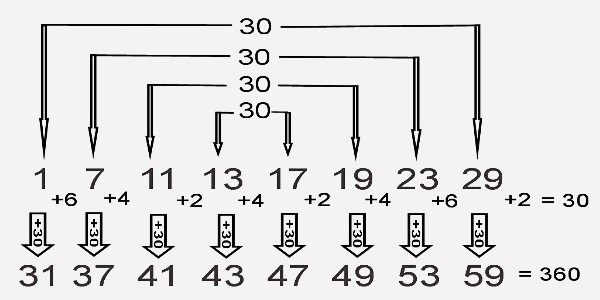Speaking of the Fibonacci number sequence, there is symmetry mirroring the above in the relationship between the terminating digits of Fibonacci numbers and their index numbers equating to members of the array populating the Prime Spiral Sieve.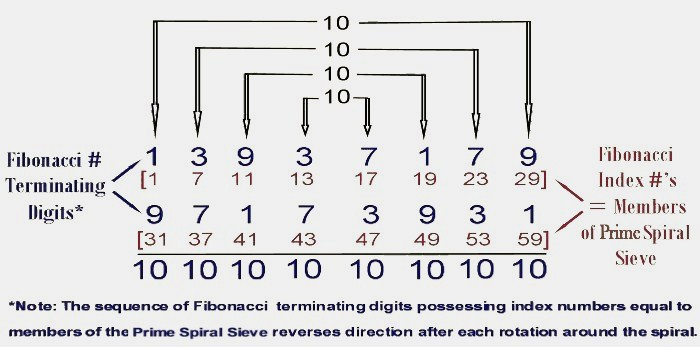These objects will then behave as a complex numbers that leads to trivial and complex roots of the 18th prime identity. 286 - (231x5)/(11x7) = 286 - 1155/77 = 286 - 15 = 200 + 71 = 271

``````  -----------------------+----+----+----+----+----+----+----+----+----+-----
True Prime Pairs Δ    |  1 |  2 |  3 |  4 |  5 |  6 |  7 |  8 |  9 | Sum
=======================+====+====+====+====+====+====+====+====+====+=====
19 → π(10)            |  2 |  3 |  5 |  7 |  - |  - |  - |  - |  - | 4th  4 x Root
-----------------------+----+----+----+----+----+----+----+----+----+-----
17 → π(20)            | 11 | 13 | 17 | 19 |  - |  - |  - |  - |  - | 8th  4 x Twin
-----------------------+----+----+----+----+----+----+----+----+----+-----
13 → π(30) → 12 (Δ1)  | 23 | 29 |  - |  - |  - |  - |  - |  - |  - |10th ←------------ 10
=======================+====+====+====+====+====+====+====+====+====+===== 1st Twin
11 → π(42)            | 31 | 37 | 41 |  - |  - |  - |  - |  - |  - |13th
-----------------------+----+----+----+----+----+----+----+----+----+----- 2nd Twin
7 → π(60) → 19 (Δ12) | 43 | 47 | 53 | 59 |  - |  - |  - |  - |  - |17th
-----------------------+----+----+----+----+----+----+----+----+----+----- 3rd Twin
5 → π(72) → 18 (Δ13) | 61 | 67 | 71 |  - |  - |  - |  - |  - |  - |20th ←------------ 20 --------
=======================+====+====+====+====+====+====+====+====+====+===== 4th Twin                |
3,2 → 18+13+12 → 43  | 73 | 79 | 83 | 89 | 97 | 101| 103| 107| 109|29th ------------→ 30 --------
=======================+====+====+====+====+====+====+====+====+====+===== bilateral 9 sums (2)+60+40=102
3,2 → 18+13+12 → 43  | 73 | 79 | 83 | 89 | 97 | 101| 103| 107| 109|29th ------------→ 30 --------
=======================+====+====+====+====+====+====+====+====+====+===== 4th Twin                |
5 → π(72) → 18 (Δ13) | 61 | 67 | 71 |  - |  - |  - |  - |  - |  - |20th ←------------ 20 --------
-----------------------+----+----+----+----+----+----+----+----+----+----- 3rd Twin
7 → π(60) → 19 (Δ12) | 43 | 47 | 53 | 59 |  - |  - |  - |  - |  - |17th
``````

The opposite direction will be made through switching beetween Linux and Windows which is proceed the old strand in the 3′ to 5′ direction, while the new strand is synthesized in the 5' to 3' direction. Here we set a remote self-host runner via WSL.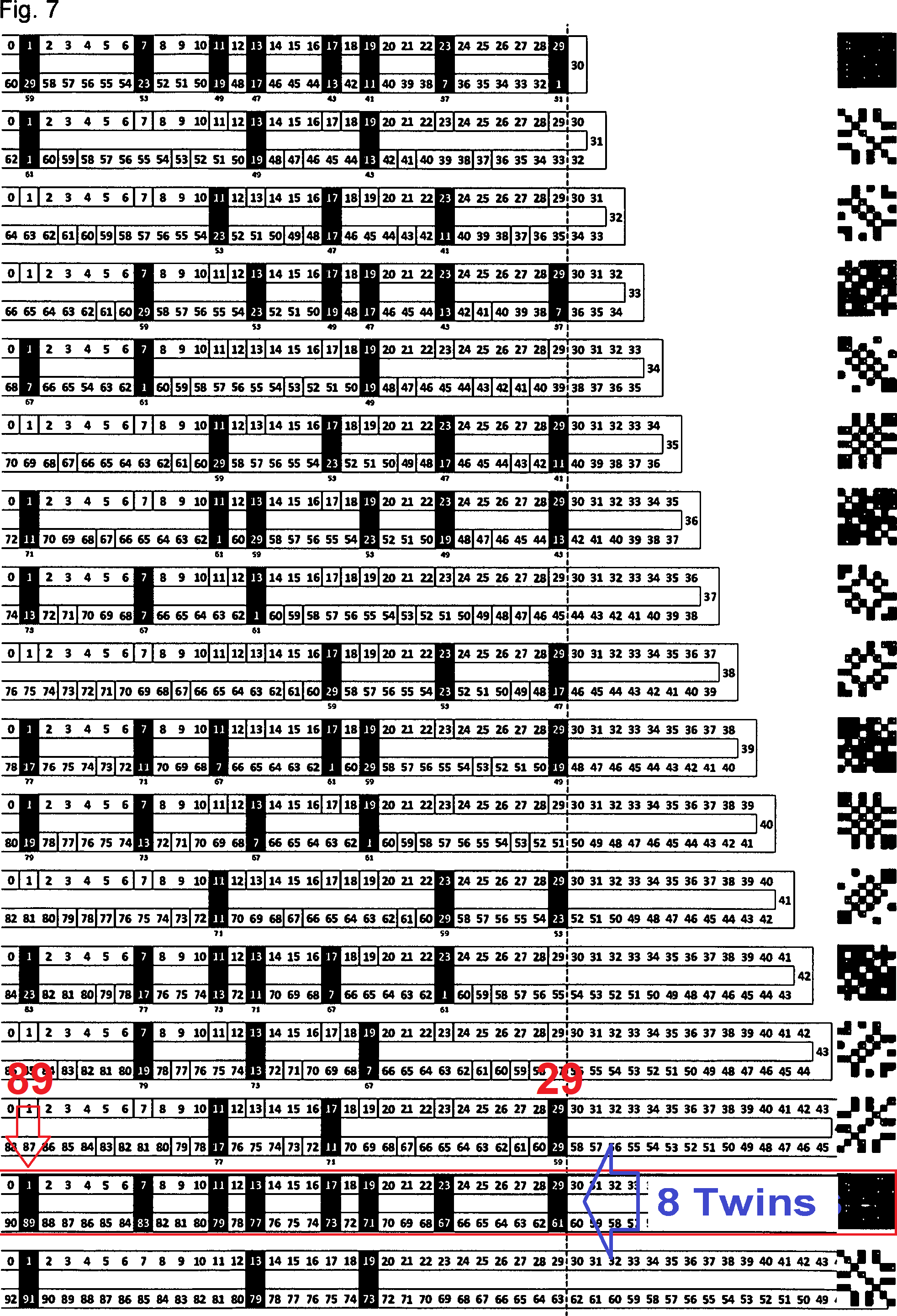The rest of primes goes to the 33's of 15th axis that holding 102 primes of (2,60,40). By the bilateral way the form will be splitted to (1,30,20). Since the base frame shall be 40 so it will be forced to form (1,30,40) of prime 71.